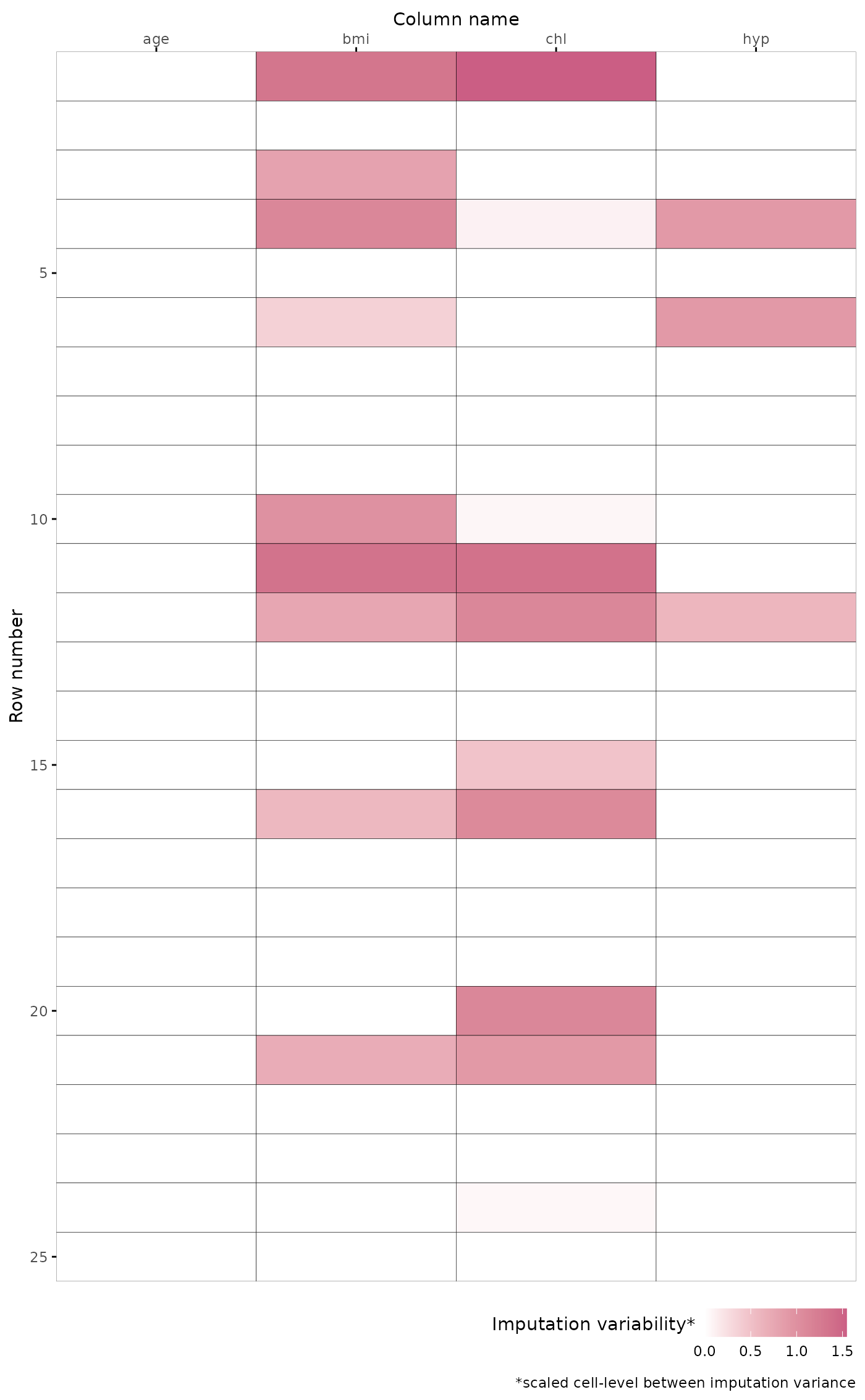This function plots the cell-level between imputation variance. The function scales the variances column-wise, without centering cf. base::scale(center = FALSE) and plots the data image as a heatmap. Darker red cells indicate more variance, lighter cells indicate less variance. White cells represent observed cells or unobserved cells with zero between imputation variance.

## Usage

plot_variance(data, grid = TRUE, caption = TRUE)

## Arguments

data

A multiply imputed object of class mice::mids.

grid

Logical indicating whether grid lines should be displayed.

caption

Logical indicating whether the figure caption should be displayed.

## Value

An object of class ggplot.

## Examples

imp <- mice::mice(mice::nhanes, printFlag = FALSE)
plot_variance(imp)